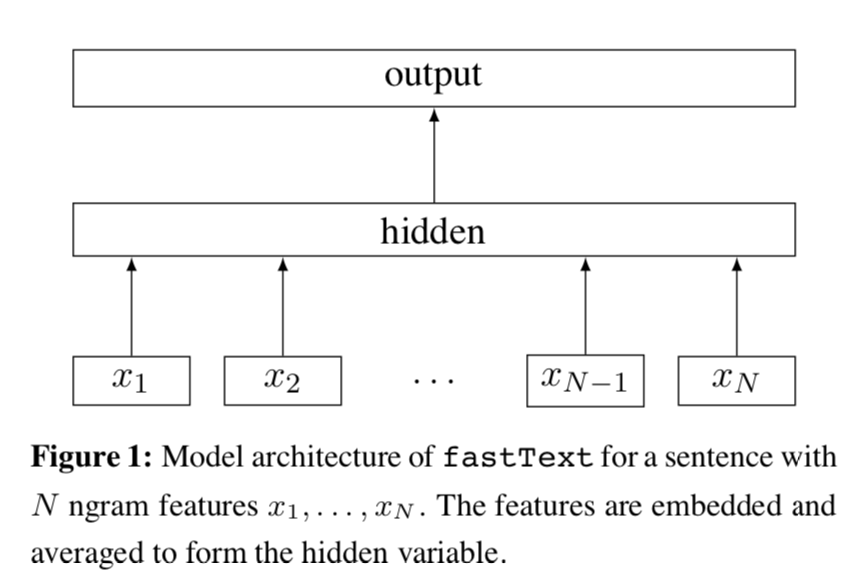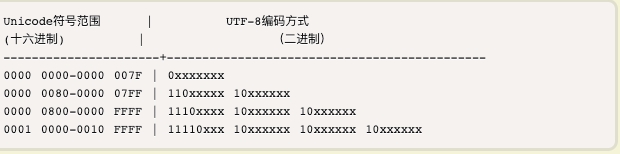# 无所不能的Embedding2 - 词向量三巨头之FastText详解

## Fasttext 分类模型Fasttext分类模型结构很直观是一个浅层的神经网络。先对文本的每个词做embedding得到$w_i$, 然后所有词的embedding做平均得到文本向量$w_{doc}$，然后经过1层神经网络对label进行预测

$$w_{doc} = \frac{1}{n}\sum_{i=1}^n w_{i} \ p = \sigma {(\beta \cdot w_{doc})} \$$

Fasttext对此的解决方法是使用hashing把n-gram映射到bucket, 相同bucket的n-gram共享一个词向量。

## Fasttext 词向量模型

Fasttext另一个模型就是词向量模型，是在Skip-gram的基础上，创新加入了subword信息。也就是把单词分解成字符串，模型学习的是字符串embedding ，单词的embedding由字符embedding求平均得到，这也是Fasttext词向量可以infer样本外单词的原因。

c++ void Dictionary::initNgrams() { for (size_t i = 0; i < size_; i++) { std::string word = BOW + words_[i].word + EOW; // 词前后加入<>用来区分单词和字符 words_[i].subwords.clear(); words_[i].subwords.push_back(i); //先把单词本身加入subword if (words_[i].word != EOS) { computeSubwords(word, words_[i].subwords); } } }

void Dictionary::computeSubwords( const std::string& word, std::vector& ngrams, std::vector* substrings) const { for (size_t i = 0; i < word.size(); i++) { std::string ngram; if ((word[i] & 0xC0) == 0x80) { continue; // 遇到10开头字节跳过，保证中文从第一个字节开始读 } for (size_t j = i, n = 1; j < word.size() && n <= args_->maxn; n++) { ngram.push_back(word[j++]); while (j < word.size() && (word[j] & 0xC0) == 0x80) { ngram.push_back(word[j++]); // 如果是中文，读取该字符的所有字节 } if (n >= args_->minn && !(n == 1 && (i == 0 || j == word.size()))) {// subword满足长度，得到hash值加入到ngrams里面 int32_t h = hash(ngram) % args_->bucket; pushHash(ngrams, h); if (substrings) { substrings->push_back(ngram); } } } } } 

• 单字节的符号，字节的第一位是0后面7位是unicode，英文的ASCII和utf-8是一样滴
• n字节的符号，第一个字节的前n位是1，后面字节的前两位一律是10，是的此10就是彼10。REF 1. P. Bojanowski, E. Grave, A. Joulin, T. Mikolov, Enriching Word Vectors with Subword Information 2. A. Joulin, E. Grave, P. Bojanowski, T. Mikolov, Bag of Tricks for Efficient Text Classification 3. A. Joulin, E. Grave, P. Bojanowski, M. Douze, H. Jégou, T. Mikolov, FastText.zip: Compressing text classification models 4. https://zhuanlan.zhihu.com/p/64960839